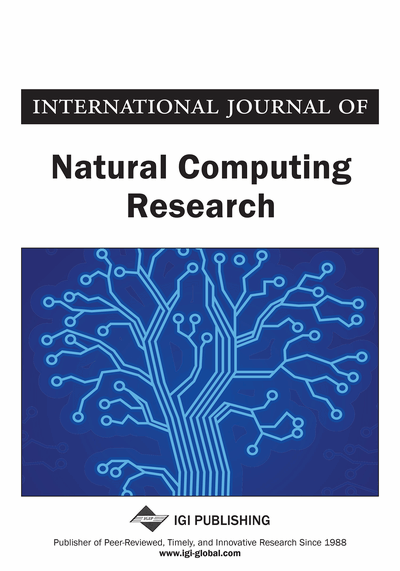# A Better Stability Control of Inverted Pendulum System Using FMINCON Based FOPID Controller Over Fractional Order Based MRAC Controller

Deep Mukherjee (KIIT University, Bhubaneswar, India), Palash Kundu (Jadavpur University, Kolkata, India) and Apurba Ghosh (UIT, Burdwan University, Bardhaman, India)
DOI: 10.4018/IJNCR.2019010102
Available
\$37.50
No Current Special Offers

## Abstract

In this article, a stability analysis on an inverted pendulum system has been approached using a fractional order PID controller and a fractional order-based model reference adaptive controller. A modified MIT rule provides an extra degree of freedom, unlike an MIT rule of MRAC controllers to stablize the pendulum angle of the inverted pendulum system which is highly unstable in nature. Here, to analyze better stability performance of the inverted pendulum over the fractional order MIT rule of MRAC controller optimal fractional order, a PID controller has been approached and FMINCON numerical optimization algorithm has been chosen to optimize the fractional order PID controller using ITSE as a scaler objective function. Next, the behaviourial characteristics of the pendulum have been compared between the FMINCON-based FOPID controllers and the fractional order MIT rule of the MRAC controller to show robust performance using an optimal FOPID controller with respect to performance indices increases time, settling time, followed by errors ISE, IAE, ITSE.
Article Preview
Top

## Introduction

In this work, design of model reference adaptive control for inverted pendulum has been suggested by normal MIT rule based on adaptation gain but this rule does not provide stability and adaptive controller designed using MIT rule is very sensitive to the amplitudes of the signals. So, the adaptive gain is usually kept small to make stable the angular movement and MIT rule has been modified as fractional order MIT rule with extra degree of freedom varying with adaptation gain to test how the performance of inverted pendulum tracks better the reference model minimizing error between them and to analyze performance nature of pendulum using fractional order rule over integer order MIT rule. Next, optimal FOPID controller has been designed using FMINCON local optimization algorithm to achieve superior performance over fractional order MIT rule of MRAC controller minimizing objective function which has been defined as a performance measure that has to be minimized. Cost includes total power consumption, integrated error and derivation from a reference value of a signal. The objective or cost function as a functional equation, which has been figured a set of points in a time series to single scalar value. FOPID controller unlike integer order PID controller has two extra degrees of freedom which help to achieve desired nature of system with better adjustment of tuning.

### Benchmark Process

The inverted pendulum is a pendulum that has its center of mass above its pivot point and has been taken as benchmark for studying control strategies. Inverted pendulum has been taken as nonlinear dynamic system including a stable resting point when pendulum is at pending position and upright position. In this work, inverted pendulum has been assumed which is shown in Figure 1.

The equation of motion for pendulum is shown in Equation (1).

(1)

Taking the Laplace transform

(2)

The parameters are given in below:

The transfer function of the actual plant is shown in Equation (3).

(3)

## Complete Article List

Search this Journal:
Reset
Open Access Articles: Forthcoming
Volume 11: 4 Issues (2022): Forthcoming, Available for Pre-Order
Volume 10: 4 Issues (2021): 3 Released, 1 Forthcoming
Volume 9: 4 Issues (2020)
Volume 8: 4 Issues (2019)
Volume 7: 4 Issues (2018)
Volume 6: 2 Issues (2017)
Volume 5: 4 Issues (2015)
Volume 4: 4 Issues (2014)
Volume 3: 4 Issues (2012)
Volume 2: 4 Issues (2011)
Volume 1: 4 Issues (2010)
View Complete Journal Contents Listing